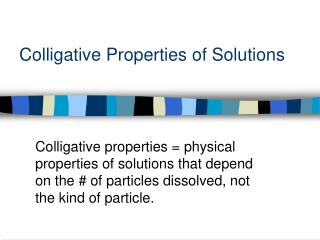# Colligative Properties of Solutions - PowerPoint PPT PresentationDownload PresentationColligative Properties of Solutions

Colligative Properties of Solutions
Download Presentation## Colligative Properties of Solutions

- - - - - - - - - - - - - - - - - - - - - - - - - - - E N D - - - - - - - - - - - - - - - - - - - - - - - - - - -
##### Presentation Transcript

1. Colligative Properties of Solutions Colligative properties = physical properties of solutions that depend on the # of particles dissolved, not the kind of particle.

2. Colligative Properties • Lowering vapor pressure • Raising boiling point • Lowering freezing point • Generating an osmotic pressure

3. Colligative Properties • Lowering vapor pressure • Raising boiling point • Lowering freezing point • Generating an osmotic pressure

4. Boiling Point Elevation • a solution that contains a nonvolatile solute has a higher boiling pt than the pure solvent; the boiling pt elevation is proportional to the # of moles of solute dissolved in a given mass of solvent.

5. Boiling Point Elevation • Tb = kbm where: Tb = elevation of boiling pt m = molality of solute kb = the molal boiling pt elevation constant • kb values are constants; see table 15-4, p. 472 (honors text) • kb for water = 0.52 °C/m

6. Ex: What is the normal boiling pt of a 2.50 m glucose, C6H12O6, solution? • “normal” implies 1 atm of pressure • Tb = kbm • Tb = (0.52 C/m)(2.50 m) • Tb = 1.3 C • Tb = 100.0 C + 1.3 C = 101.3 C

7. Ex: How many grams of glucose, C6H12O6, would need to be dissolved in 535.5 g of water to produce a solution that boils at 101.5°C? • Tb = kbm • 1.5C= (0.52 C/m)(m) • m = 2.885

8. Freezing/Melting Point Depression • The freezing point of a solution is always lower than that of the pure solvent.

9. Freezing/Melting Point Depression • Tf = kfm where: Tf = lowering of freezing point m = molality of solute kf = the freezing pt depression constant • kf for water = 1.86 °C/m • kf values are constants; see table 15-5, p. 474 (honors text)

10. Ex: Calculate the freezing pt of a 2.50 m glucose solution. • Tf = kfm • Tf = (1.86 C/m)(2.50 m) • Tf = 4.65 C • Tf = 0.00C - 4.65 C = -4.65C

11. Ex: When 15.0 g of ethyl alcohol, C2H5OH, is dissolved in 750 grams of formic acid, the freezing pt of the solution is 7.20°C. The freezing pt of pure formic acid is 8.40°C. Determine Kf for formic acid. Tf = kfm 1.20C= (kf)( 0.4348 m) kf = 2.8 C/m

12. Ex: An antifreeze solution is prepared containing 50.0 cm3 of ethylene glycol, C2H6O2, (d = 1.12 g/cm3), in 50.0 g water. Calculate the freezing point of this 50-50 mixture. Would this antifreeze protect a car in Chicago on a day when the temperature gets as low as –10° F? (-10 °F = -23.3° C) Tf = kfm Tf = (1.86C/m)(18.06 m) Tf = 33.6 C Tf = 0 C – 33.6 C = -33.6 C YES!

13. Electrolytes and Colligative Properties • Colligative properties depend on the # of particles present in solution. • Because ionic solutes dissociate into ions, they have a greater effect on freezing pt and boiling pt than molecular solids of the same molal conc.

14. Electrolytes and Colligative Properties • For example, the freezing pt of water is lowered by 1.86°C with the addition of any molecular solute at a concentration of 1 m. • Such as C6H12O6, or any other covalent compound • However, a 1 m NaCl solution contains 2 molal conc. of IONS. Thus, the freezing pt depression for NaCl is 3.72°C…double that of a molecular solute. • NaCl  Na+ + Cl- (2 particles)

15. Electrolytes - Boiling Point Elevation and Freezing Point Depression The relationships are given by the following equations: • Tf = kf ·m·norTb = kb·m·n Tf/b = f.p. depression/elevation of b.p. m = molality of solute kf/b = b.p. elevation/f.p depression constant n = # particles formed from the dissociation of each formula unit of the solute

16. Ex: What is the freezing pt of:a) a 1.15 m sodium chloride solution? • NaCl  Na+ + Cl- n=2 • Tf = kf·m·n • Tf = (1.86 C/m)(1.15 m)(2) • Tf = 4.28 C • Tf = 0.00C - 4.28 C = -4.28C

17. Ex: What is the freezing pt of:b) a 1.15 m calcium chloride solution? • CaCl2  Ca2+ + 2Cl- n=3 • Tf = kf·m·n • Tf = (1.86 C/m)(1.15 m)(3) • Tf = 6.42 C • Tf = 0.00C – 6.42 C = -6.42C

18. Ex: What is the freezing pt of:c) a 1.15 m calcium phosphate solution? • Ca3(PO4)2  3Ca2+ + 2PO43- • n=5 • Tf = kf·m·n • Tf = (1.86 C/m)(1.15 m)(5) • Tf = 10.7 C • Tf = 0.0C – 10.7 C = -10.7C

19. Determining Molecular Weights by Freezing Point Depression

20. Ex: A 1.20 g sample of an unknown molecular compound is dissolved in 50.0 g of benzene. The solution freezes at 4.92°C. Determine the molecular weight of the compound. The freezing pt of pure benzene is 5.48°C and the Kf for benzene is 5.12°C/m. • Tf = 0.56°C • Tf = kf·m • 0.56°C = (5.12°C/m)(m) • m = 0.1094

21. Ex: A 37.0 g sample of a new covalent compound was dissolved in 200.0 g of water. The resulting solution froze at –5.58°C. What is the molecular weight of the compound? • Tf = 5.58°C • Tf = kf·m • 5.58°C = (1.86°C/m)(m) • m = 3.00 m